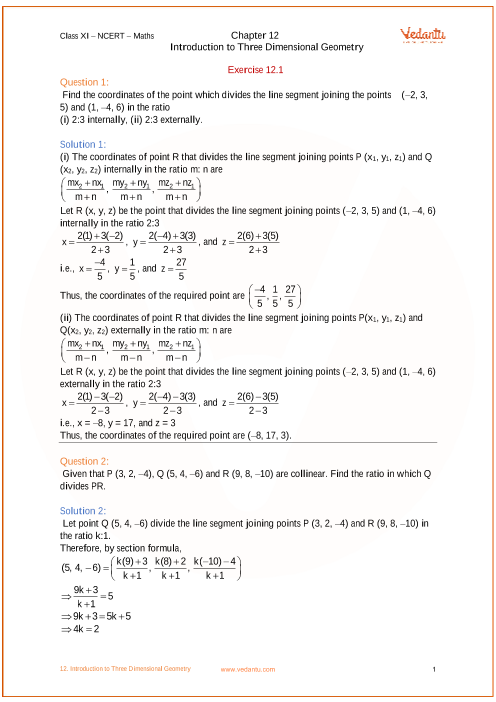NCERT Solutions for Class 11 Maths Chapter 12 Introduction to Three Dimensional Geometry (Ex 12.1) Exercise 12.1

NCERT Solutions for Class 11 Maths Chapter 12 Introduction to Three Dimensional Geometry (Ex 12.1) Exercise 12.1

Free PDF download of NCERT Solutions for Class 11 Maths Chapter 12 Exercise 12.1 (Ex 12.1) and all chapter exercises at one place prepared by expert teacher as per NCERT (CBSE) books guidelines. Class 11 Maths Chapter 12 Introduction to Three Dimensional Geometry Exercise 12.1 Questions with Solutions to help you to revise complete Syllabus and Score More marks. Register and get all exercise solutions in your emails.